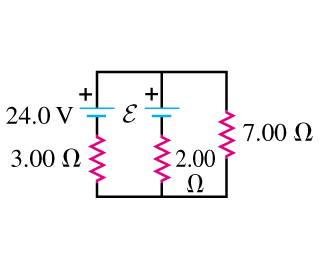# Parallel circuits, Emfs and kirchoff's laws

• Prodigium
In summary, the current through the 7Ω resistor must be 1.79A in order for the emf in the figure to be equal to the current through the 3.00 Ohm resistor. Each emf source has negligible internal resistance, so the equation for the outer loop is valid. However, the left and right hand loops have problems because the voltage drops and rises across the resistors are not consistent.f

## Homework StatementWhat must the emf ε in the figure be in order for the current through the 7Ω resistor to be 1.79A ? Each emf source has negligible internal resistance.

## Homework Equations

V=IR
Kirchoffs junc and loop rules

## The Attempt at a Solution

I put that the outer loop would be 24V +3*I = 7*1.79
and from there solved for I
i got that I would be -5.02A
I then said that because the current I split at the top middle junc the current I{2} down the centre line was I{2}=I-I{1}
and basically used V=IR to solve for ε which i got to be -13.62V

but i can't help but feel I've done it wrong, and in the textbook there is no answers can anyone help? much appreciated

First, check the equation that you wrote for the outer loop. If the current is assumed to be flowing clockwise then there should be voltage DROPS across both the 3.00 and 7.00 Ohm resistors.

You might want to consider using nodal analysis (KCL). After all, there's only one node and you already know it's voltage: 1.79 x 7.00. You'll end up with a single equation with one unknown.

Okay so I got (using kirchhoffs lecturer says we have to use kirchhoffs laws)
outer loop :-
24-3I-7I2=0
left hand loop :-
24+ε-3I-2I1=0
right hand loop:-
-ε+2I1-7I2=0

i went on to solve and didnt get a consisted answer... (when put back into equations to check)
are these equations on the right line? I am not the best at circuits.

Okay so I got (using kirchhoffs lecturer says we have to use kirchhoffs laws)
outer loop :-
24-3I-7I2=0
left hand loop :-
24+ε-3I-2I1=0
right hand loop:-
-ε+2I1-7I2=0

i went on to solve and didnt get a consisted answer... (when put back into equations to check)
are these equations on the right line? I am not the best at circuits.

I see that you're having trouble keeping the signs straight for the voltage rises and drops whe you "walk" around a loop. Your equation for the outer loop looks okay, but the other two have some problems.

Take your figure and draw in the assumed directions of each of the currents (I, I1, I2). Now do the left loop again, walking around the loop clockwise starting at the - terminal of the battery. The next component you come to is the source ε. As you walk over it, will there be a voltage rise or a voltage drop?

Thanks so much i have a consistant answer now :D and i am more confident with circuits.